# Selina Concise Physics Class 10 ICSE Solutions Force

## Selina Concise Physics Class 10 ICSE Solutions Force

APlusTopper.com provides step by step solutions for Selina Concise ICSE Solutions for Class 10 Physics Chapter 1 Force. You can download the Selina Concise Physics ICSE Solutions for Class 10 with Free PDF download option. Selina Publishers Concise Physics for Class 10 ICSE Solutions all questions are solved and explained by expert teachers as per ICSE board guidelines.

Selina ICSE Solutions for Class 10 Physics Chapter 1 Force

Exercise 1(A)

Solution 1.

(a) When the body is free to move it produces translational motion.
(b) When the body is pivoted at a point, it produces rotational motion.

Solution 2.

The moment of force is equal to the product of the magnitude of the force and the perpendicular distance of the line of action of force from the axis of rotation.
S.I. unit of moment of force is Newton metre (Nm).

Solution 3.

Moment of a force is a vector.

Solution 4.

Moment of force about a point depends on the following two factors:

1. The magnitude of the force applied and,
2. The distance of line of action of the force from the axis of rotation.

Solution 5.

When the body is pivoted at a point, the force applied on the body at a suitable point rotates the body about the axis passing through the pivoted point.
The direction of rotation can be changed by changing the point of application of force. The given figure shows the anticlockwise and clockwise moments produced in a disc pivoted at its centre by changing the point of application of force F from A to B.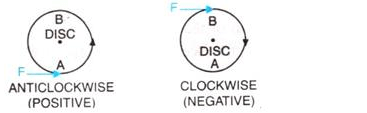Solution 6.

Moment of force about a given axis = force x perpendicular distance of force from the axis of rotation.

Solution 7.

Moment of force depends on the distance of line of action of the force from the axis of rotation. Decreasing the perpendicular distance from the axis reduces the moment of a given force.

Solution 9.

If the turning effect on the body is anticlockwise, moment of force is called anticlockwise moment and it is taken as positive while if the turning effect on the body is clockwise, moment of force is called clockwise moment and is taken negative.

Solution 10.

It is easier to open a door by applying the force at the free end of it because larger the perpendicular distance , less is the force needed to turn the body.

Solution 11.

The stone of hand flour grinder is provided with a handle near its rim so that it can be rotated easily about the iron pivot at its centre by a small force applied at the handle.

Solution 12.

It is easier to turn the steering wheel of a large diameter than that of a small diameter because less force is applied on steering of large diameter which is at a large distance from the centre of rim.

Solution 13.

A spanner (or wrench) has a long handle to produce larger turning moment so that nut can easily be turned with a less force.

Solution 14.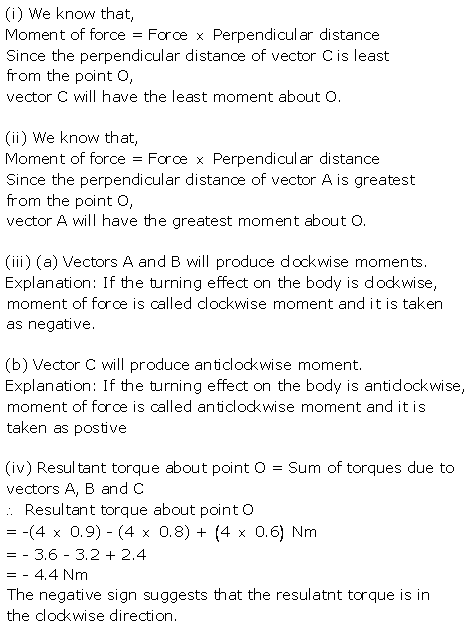Solution 15.Solution 16.

(a) Resultant force acting on the body = F-F=0 moment of forces = 0 i.e., no motion of the body
(b) The forces tend to rotate the body about the mid-point between two forces, Moment of forces= Fr

Solution 17.At A and B, two equal and opposite forces each of magnitude F are applied. The two forces rotate the bar in anticlockwise direction.

Solution 18.

Two equal and opposite parallel forces not acting along the same line, form a couple. A couple is always needed to produce the rotation. For example, turning a key in a lock and turning a steering wheel.

Solution 19.

The moment of a couple is equal to the product of the either force and the perpendicular distance between the line of action of both the forces. S.I unit of moment of couple is Nm.

Solution 20.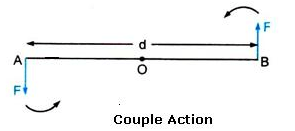At A and B, two equal and opposite forces each of magnitude F are applied. The two forces rotate the bar in anticlockwise direction. The perpendicular distance between two forces is AB which is called the couple arm.
Moment of force F at the end A
= F x OA(anticlockwise)
Moment of force F at the end B
= F x OB(anticlockwise)
Total moment of couple =F x OA + F x OB
= F x (OA +OB)= F x AB
= F x d(anticlockwise)
=Either force x perpendicular distance between the two forces (or couple arm)
Thus, Moment of couple = Force x Couple arm

Solution 21.

When a number of forces acting on a body produce no change in its state of rest or of motion, the body is said to be in equilibrium.

Solution 22.

(i) When a body remains in the state of rest under the influence of the applied forces, the body is in static equilibrium. For example a book lying on a table is in static equilibrium.
(ii) When a body remains in the same state of motion (translational or rotational), under the influence of the applied forces, the body is said to be in dynamic equilibrium. For example, a rain drop reaches the earth with a constant velocity is in dynamic equilibrium.

Solution 23.

For a body to be in equilibrium:

1. The resultant of all the forces acting on the body should be equal to zero.
2. The resultant moment of all the forces acting on the body about the point of rotation should be zero.

Solution 24.

According to the principle of moments, if the algebraic sum of moments of all the forces acting on the body about the axis of rotation is zero, the body is in equilibrium. A physical balance (or beam balance) works on the principle of moments.

Solution 25.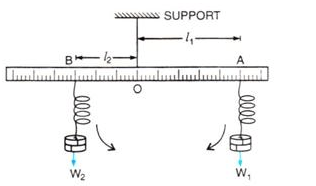Solution 1 (MCQ).

The moment of a force about a given axis depends on both on the force and its perpendicular distance from the axis.
Hint: Moment of force = Force x Perpendicular distance

Solution 2 (MCQ).

The body will have rotational as well as translational motion.

Numericals

Solution 1.

Moment of force= force x perpendicular distance of force from point O
Moment of force= F x r
5Nm= 10 x r
R= 5/10 =0.5 m

Solution 2.

Length, r=10cm =0.1m
F= 5N
Moment of force= F x r= 5 x 0.1 = 0.5 Nm

Solution 3.

Given , F= 2N
Diameter=2m
Perpendicular distance between B and O =1m
(i)Moment of force at point O
= F x r
= 2 x 1=2Nm (clockwise)
(ii)Moment of force at point A= F x r
= 2 x 2=4Nm (clockwise)

Solution 4.

Given AO=2m and OB=4m

(i) Moment of force F1(=5N) at A about the point O
=F1 x OA
=5 x 2= 10Nm (anticlockwise)

(ii) Moment of force F2(=3N) at B about the point O
= F2 x OB
=3 x 4=12 Nm(clockwise)

(iii) Total moment of forces about the mid-point O
= 12- 10=2Nm(clockwise)

Solution 5.

Given, AB=4m hence, OA=2m and OB =2m
Moment of force F(=10N) at A about the point O
= F x OA= 10 x 2= 20Nm (clockwise)
Moment of force F (=10N) at point B about the point O
= F x OB= 10 x 2 =20Nm (clockwise)
Total moment of forces about the mid-point O=
=20 +20= 40Nm(clockwise)

Solution 6.

(i) Perpendicular distance of point A from the force F=10 N at B is 0.5m , while it is zero from the force F=10N at A
Hence, moment of force about A is
= 10 N x 0.5m=5Nm(clockwise)

(ii) Perpendicular distance of point B from the force F=10 N at A is 0.5m, while it is zero from the force F=10N at B
Hence, moment of force about B is
= 10 N x 0.5m=5Nm(clockwise)

(iii) Perpendicular distance of point O from either of the forces F=10N is 0.25 m
Moment of force F(=10N) at A about O= 10N x 0.25m
=2.5Nm(clockwise)
And moment of force F(=10N) at B about O
=10N x 0.25m=2.5Nm(clockwise)
Hence, total moment of the two forces about O
=0.25 + 0.25=5Nm (clockwise)

Solution 7.Solution 8.
Let the 50gf weight produce anticlockwise moment about the middle point of metre rule .i.e, at 50cm.
Let a weight of 100gf produce a clockwise moment about the middle point. Let its distance from the middle be d cm. Then,
according to principle of moments,
Anticlockwise moment = Clockwise moment
50gf x 50 cm=100gf x dSolution 9.

(i) Weight mg (W) of rule produces an anti-clockwise moment about the knife edge O. In order to balance it, 20gf must be suspended at the end B to produce clockwise moment about the knife edge O.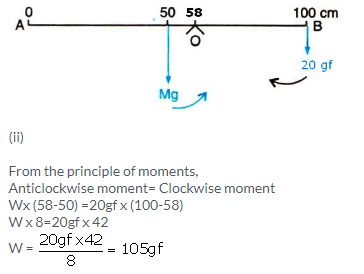Solution 10.

Anticlockwise moment= 40gf x 40 cm
Clockwise moment= 80gf x d cm
From the principle of moments,
Anticlockwise moment= Clockwise moment
40gf x 40 cm =80gf x d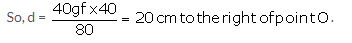Solution 11.

(i) Anticlockwise moment= 40gf x (50-10)cm
=40gf x 40cm=1600 gf x cm
Clockwise moment= 20gf x (90- 50) =20gf x 40cm
=800 gf x cm
Anticlockwise moment is not equal to clockwise moment. Hence the metre rule is not in equilibrium and it will turn anticlockwise.

(ii) To balance it, 40gf weight should be kept on right hand side so as to produce a clockwise moment about the middle point. Let its distance from the middle be d cm. Then,
clockwise moment= 20gf x 40cm + 40gf x d cm
From the principle of moments,
Anticlockwise moment= Clockwise moment
40 gf x 40 cm= 20gf x 40 + 40 x d cm
1600-800=40gf x dcmSolution 12.

From the principle of moments,
Anticlockwise moment= Clockwise moment
20kgf x 2m =40kgf x d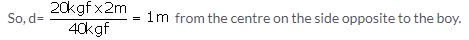Solution 13.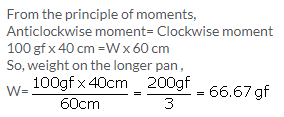Solution 14.

(i) Total anticlockwise moment about O
= 150gf x 40 cm=6000gf cm

(ii) Total clockwise moment about O,
=250gf x 20 cm= 5000gf cm

(iii) The difference of anticlockwise and clockwise moment= 6000- 5000= 1000gf cm

(iv) From the principle of moments,
Anticlockwise moment= Clockwise moment
To balance it, 100gf weight should be kept on right hand side so as to produce a clockwise moment about the O. Let its distance from the point O be d cm. Then,
150gf x 40 cm=250gf x 20 cm +100gf x d
6000gf cm= 5000gf cm + 100gf x d
1000gf cm =100 gf x d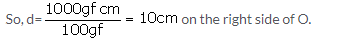Solution 15.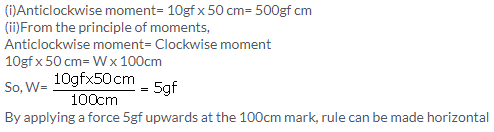Solution 16.Solution 17.

(i) From the principle of moments,
Clockwise moment= Anticlockwise moment
100g x (50-40) cm= mx(40-20) cm
100g x 10 cm = m x 20 cm = m =50 g

(ii) The rule will tilt on the side of mass m (anticlockwise), if the mass m is moved to the mark 10cm.

(iii) Anticlockwise moment if mass m is moved to the mark 10 cm= 50g x (40-10)cm =50 x 30=1500g cm
Clockwise moment=100g x (50-40) cm= 1000g cm
Resultant moment= 1500g cm -1000g cm= 500g cm (anticlockwise)

(iv) From the principle of moments,
Clockwise moment= Anticlockwise moment
To balance it, 50g weight should be kept on right hand side so as to produce a clockwise moment .Let its distance from fulcrum be d cm. Then,
100g x (50-40) cm + 50g x d =50g x (40-10)cm
1000g cm + 50g x d =1500 g cm
50 g x d= 500g cm
So, d =10 cm
By suspending the mass 50g at the mark 50 cm, it can be balanced.

Exercise 1(B)

Solution 1.

Centre of gravity is the point about which the algebraic sum of moments of weights of particles constituting the body is zero and the entire weight of the body is considered to act at this point.

Solution 2.

Yes, the centre of gravity can be situated outside the material of the body. For example, centre of gravity of ring.

Solution 3.

The position of centre of gravity of a body of given mass depends on its shape i.e., on the distribution of mass in it. For example: the centre of gravity of a uniform wire is at its mid-point. But if this wire is bent into the form of a circle, its centre of gravity will then be at the centre of circle.

Solution 4.

The position of centre of gravity of a
(a) rectangular lamina is at the point of intersection of its diagonals.
(b) cylinder is at the mid point on the axis of cylinder.

Solution 5.

(a) Centre of gravity of a triangular lamina is situated at the point of intersection of its medians.
(b) Centre of gravity of a circular lamina is situated at the centre of circular lamina.

Solution 6.

Centre of gravity of a uniform ring is situated at the centre of ring.

Solution 7.Solution 8.

Take a triangular lamina. Make three fine holes at a, b, c near the edge of triangular lamina. Now suspend the given lamina along with a plumb line from hole ‘a’. Check that the lamina is free to oscillate about the point of suspension. When lamina has come to rest, draw straight line ad along the plumb line. Repeat the experiment by suspending the lamina through hole ‘b’ and then through hole ‘c’ for which we get straight lines be and cf respectively. It is noticed that the lines ad, be and cf intersect each other at a common point G which is the position of centre of gravity of triangular lamina i.e. the point of intersection of medians.Solution 9.

(i) False. The position of centre of gravity of a body of given mass depends on its shape i.e., on the distribution of mass in it.
(ii) True.

Solution 10.Solution 11.Solution 1 (MCQ).

At its geometrical centre

Exercise 1(C)

Solution 1.

When a particle moves with a constant speed in a circular path, its motion is said to be the uniform circular motion. For example : Revolution of earth around sun is an example of uniform circular motion.

Solution 2.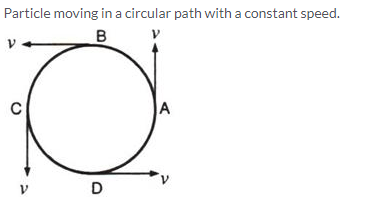Solution 3.

Yes, uniform circular motion has an accelerated motion with a constant speed.

Solution 4.

Motion of a cyclist on a circular track is an example of motion in which speed remains uniform, but the velocity changes.

Solution 5.

When the object moves in a circular path with uniform speed, it means that its magnitude of velocity does not change, only its direction changes continuously. Hence, it is considered as uniformly accelerated motion.

Solution 6.

 Uniform linear motion Uniform circular motion The body moves along a straight line. The body moves along a circular path. Speed and direction both remain constant. Speed is constant, but direction changes continuously. It is not an accelerated motion. It is an accelerated motion.

Solution 7.

Centripetal force is required for circular motion. It is always directed towards the centre of circle.

Solution 8.

Force acting on a body which is in circular motion is called centripetal force. It acts towards the centre of circular path.

Solution 9.

A planet moves around the sun in a nearly circular path for which the gravitational force of attraction on the planet by the sun provides the necessary centripetal force required for circular motion.

Solution 10.

(a) They act in opposite directions.
(b) No, centrifugal force is not the force of reaction of centripetal force.

Solution 11.

No, centrifugal force is a fictitious force.

Solution 12.

a. On standing outside the disc, we find that the pebble is moving on a circular path. On standing at the centre of the disc, we find that the pebble is stationary placed just in front of us.

Solution 13.

Force of tension in the thread provides the centripetal force.

Solution 15.

(a) False
(b) True
(c) True
(d) False

Solution 1 (MCQ).

Speed
Hint: Speed is scalar but velocity and acceleration are vector quantities. So, speed remains constant but velocity and acceleration change with the change in direction, and in circular motion the direction of motion changes at every point.

More Resources for Selina Concise Class 10 ICSE Solutions